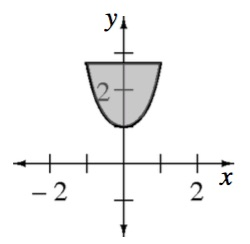### Home > APCALC > Chapter 8 > Lesson 8.4.1 > Problem8-140

8-140.

Calculate the volume of the solid constructed as follows: The base of the solid is the region is formed by the curve $y = e^{x^2}$ and the line $y = e$, while the cross-sections perpendicular to the $y$-axis are squares.

Start by sketching the base of the solid. Notice the $x$- and $y$- coordinates where the functions intersect. Also notice the bottom-most point on the solid. Visualize the square cross-sections that will stand up upon this base, making a 3D figure. Each cross-section is perpendicular to the $y$ axis, which means they will be horizontally aligned. At the bottom, near $y = 1$, the squares will be very small. As you move up, they will gradually get larger. The largest square, at $y = e$, will have a side length of $2$.

The bounds: Since the squares are horizontal, the bounds will be $y$-values. What are the lowest and the highest $y$-values?The integrand:
Since the squares are horizontal, the integrand must be written in terms of $y$.
In other words, solve for $x$.
$y = e^{x^2}$
$\ln(y) = x^2\ln(e)$

$x=\sqrt{\ln(y)}$

Careful! Observe that, because of symmetry across the $y$-axis, $x$ represents just half of the side-length of each square.

$\text{side }=2\sqrt{\ln(y)}$

The integral:
Put it all together.

$\text{volume by cross-sections }=\int_{y=a}^{y=b}(\text{area})dy$